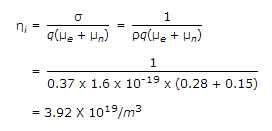# Electronics and Communication Engineering - Exam Questions Papers

21.

In the following network, the switch is closed at t = 0 and the sampling starts from t = 0. The sampling frequency is 10Hz.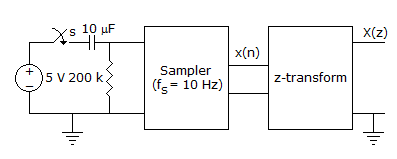The samples x(n) (n = 0, 1, 2, ...) are given by

 A. 5(1 - e-0.05n) B. 5e-0.05n C. 5(1 - e-5n) D. 5e-5n

Explanation:

Initial voltage across R = 5Vn

Final voltage across R = 0V

Thus VR = 5.0-0.5t

When we sample this at f = 102

We get x(n) =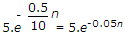.

22.

Find 'X' in the circuit below:
f1(A, B, C, D) = Σ(6, 7, 13, 14);
f2(A, B, C, D) = Σ(3, 6, 7);
f3(A, B, C, D) = Σ(5, 6, 7, 14, 15)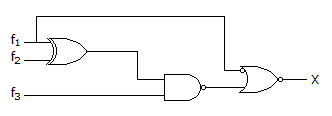A. ∏(0, 1, 2, 3, 4, 5, 6, 7, 8, 9, 10, 11, 12, 13, 15) B. 0 C. Σ(14) D. 1

Explanation:

f1(A, B, C, D) = ∑(6, 7, 13, 14)

f2(A, B, C, D) = ∑(3, 6, 7)

f1f2 = ∑(3, 13, 14)

f3 x (f1f2) = ∑(14)= Y

X = f1 x Y = ∑(14).

23.

In the following circuit , the comparator output in log: "I" if V1 > V2 and is logic "0" otherwise. The D/A conversion is done as per the relations VDAC =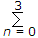2n-2 Volts, where b3 (MSB), b2, b1 and b0 (LSB) are the counter outputs. The counter starts from the clear state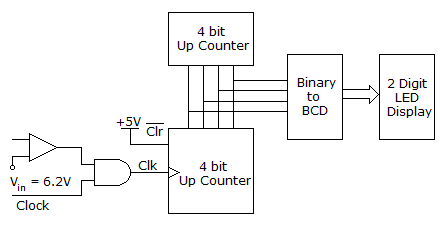The stable reading of the LED display is

 A. 06 B. 07 C. 12 D. 13

Explanation: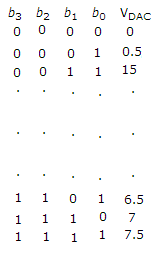Using the given VDAC relation we can get tc

Thus stable reading of LED is 6.5 x 2 = 13.

24.

The transfer characteristic for the precision rectifier circuit shown below is (assume ideal OP-AMP and practical diodes)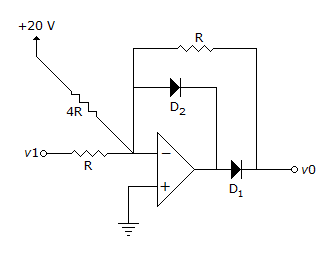A.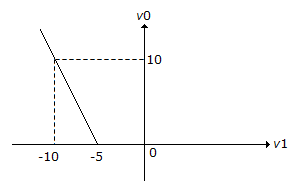B.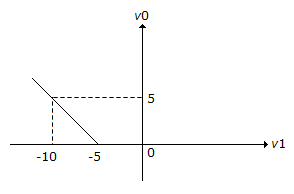C.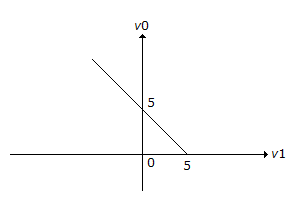D.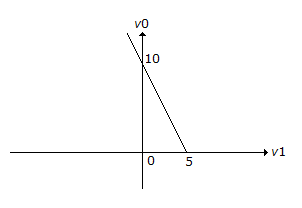Explanation:

When Vi = - 10 V0 = 5.

25.

If the intrinsic resistivity at 300°K is 0.37 ohm-m and the electron and hole mobilities at 300°K are 0.28 and 0.15 m2/volt-sec, then the intrinsic carrier concentration of Germanium is __________ .

 A. 2.92 x 1019/m3 B. 2.92 x 10-19/m3 C. 1.92 x 1019/m3 D. 3.92 x 1019/m3